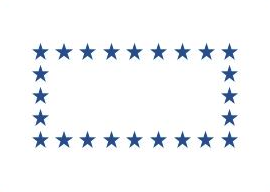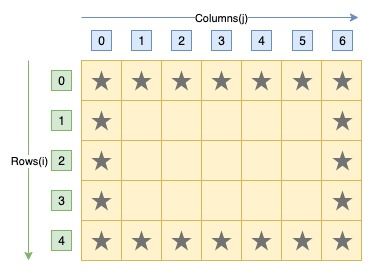# C Program to Print Hollow Rectangle Star Pattern

Here is a C program to print hollow rectangular star pattern of n rows and m columns. For a hollow rectangular star pattern of 4 rows and 12 columns. Program's output should be:#### Required Knowledge

Algorithm to print hollow rectangular star pattern using loop
The algorithm of this pattern is similar to rectangular star pattern except here we have to print stars of first and last rows and columns only. At non-boundary position, we will only print a space character.
• Take the number of rows(N) and columns(M) of rectangle as input from user using scanf function.
• We will use two for loops to print empty rectangular star pattern.
• Outer for loop will iterate N times. In each iteration, it will print one row of pattern.
• Inside inner for loop, we will add a if statement check to find the boundary positions of the pattern and print star (*) accordingly.

Here is the matrix representation of the hollow rectangle star pattern. The row numbers are represented by i whereas column numbers are represented by j.## C program to print hollow rectangular star pattern

```#include<stdio.h>

int main(){
int rows, cols , i, j;

printf("Enter rows and columns of rectangle\n");
scanf("%d %d", &rows, &cols);

/* Row iterator for loop */
for(i = 0; i < rows; i++){
/* Column iterator for loop */
for(j = 0; j < cols; j++){
/* Check if currely position is a boundary position */
if(i==0 || i==rows-1 || j==0 || j==cols-1)
printf("*");
else
printf(" ");
}
printf("\n");
}
return 0;
}
```
Output
```Enter rows and columns of rectangle
4 12
************
*          *
*          *
************
```

Related Topics
 C program hollow square pattern C program rectangle star pattern C program hollow diamond star pattern C program hollow pyramid star pattern C program binary rectangle pattern C program multiplication table triangle pattern C program heart shape star pattern C program palindrome triangle pattern C program diamond star pattern List of all C pattern programs